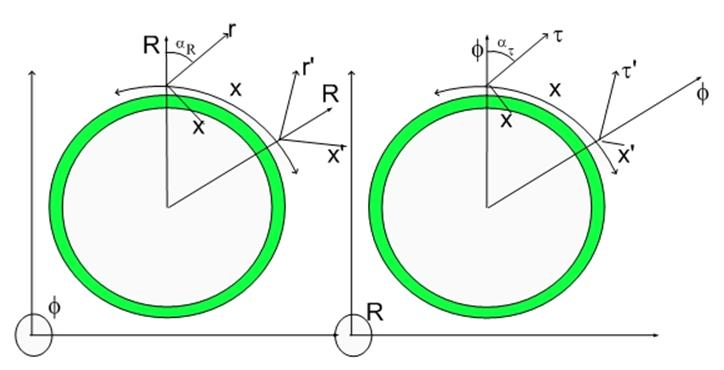Anonymous said...
are all your factors constants or functional approximations?
where is your evidence to confirm their definition for each factor, time, time change according to the history of time, what is space?
Is it constant or variable, or constant/variable, or other...
Thanks for the question. Every so often someone asks a kind of general question that permits me to review the whole theory and in many times, to find a better way to explain my ideas..:)

The theory does not have factors other than the Planck's constant, which relates 3D Mass (inertial mass) to a dilaton projection wavelength and the speed of light.

The theory reproduces the laws of physics and in doing so introduces two new constitutive parameters (elasticity anisotropy and elasticity).  These parameters can be tested by looking at the predicted gravitational wave frequency and by measuring phase match angles of nuclear reactions.

There are two more parameters (dephasings associated with transmutation notes, explained further in this blog), that are associated with the time it takes for an electron (proton) to rotate 90 degrees in the 3D space.

They differ from constants in other theories since they have a very simple and physical meanings and come in a very small number.  These six constants are the minimum information one needs to explain the whole Universe.
-----------------------------------------------------------------
The fundamental components of this theory are :
1. Particles are modeled as dilators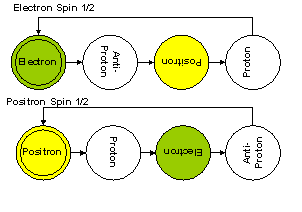Dimensional notes associated with Electron and Positron. Vertical letters indicates perpendicularity with respect to our 3D Universe
2. Dilators are coherences between stationary deformation states of a local metric.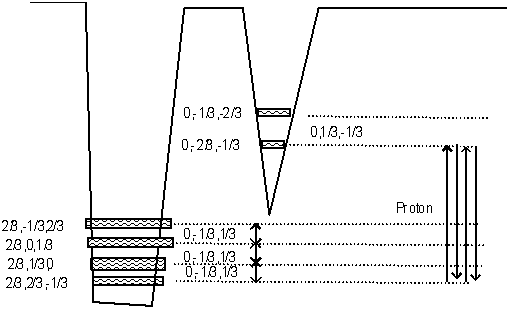Lower (higher) state is associated with Proton(Electron). The initial state (in phase with the 3D Universe) defines the particle nature (proton, antiproton,electron or positron).
3. The Hypergeometrical Universe topology (3D Universe is a lightspeed expanding hyperspherical shockwave)
4. Dilators are thin along the radial direction and spin at half the speed of tunneling, that is, when the wavefunction describing the dilator moves from one shape to another (180 degrees), the dilator profile rotates by 90 degrees.
5. Pseudo-time quantization results from item 4). Interaction only occurs when the dilator has a footprint on the 3D Universe.
6. Dilators motion along the 4D spatial manifold obeys the Quantum Lagrangian Principle which states that dilators always dilate in phase with surrounding dilaton field.
7. Items 6, 5 and the embedding of the 3D lightspeed expanding hyperspherical shockwave Universe within a 4D spatial manifold allows for the uncertainty that creates Quantum Mechanical behavior. This means that the Hypergeometrical Universe provides the reasons for the observed Quantum Mechanics.
8. The two cross-sections shown at the top of this and almost any other posting here, shows that the Hypergeometrical Universe contains an Absolute Time, a preferential direction but the absolute time cannot be used to time anything other than the Universe expansion. The preferential direction cannot be seen within the 3D expanding Hypersphere where we live.

Dilators motion along the 4D spatial manifold obeys the Quantum Lagrangian Principle which states that dilators always dilate in phase with surrounding dilaton field.At any de Broglie step of the Hypergeometrical Universe expansion (one step is equal to the Compton wavelength of a Hydrogen atom), the dilator has to move itself by distance x to satisfy the Quantum Lagrangian Principle, that is, to dilate in phase with the surrounding field.

In this picture, I show two angles. Alpha1 is the arctan(x/Lambda1), where Lambda1 is the Compton Wavelength of a Hydrogen atom (around 10^-15meters).

If you are a charged fundamental dilator (non spin zero dilator), your k-vector is now along that new direction. If you are a neutral particle (e.g. a hydrogen atom or a neutron), you will also move yourself by distance x, but your k-vector will only point along angle alpha0 or thereabouts. Angle Alpha0 is arctan(x/(R0+lambda1), where R0 is the radius of the Universe (equal to 21.72 billion light years).

The Universe is actually 21.72 Billion Years old, by which I mean that this radius is consistent with the Pioneer Anomaly (it was derived from the Pioneer Anomaly observations) and explains everything in Cosmology.  There is another way to calibrate this radius but I would need the redshift data for all the Stellar Candles.  That would be an independent measure of the age/radius of the Universe.

This difference in change of direction rate (the change in direction happens at each de Broglie step of the expansion) explains the difference in strength between electromagnetic and gravitational forces. This means that the "force" represented here by the dilaton field and the Quantum Lagrangian Principle is the same.  What differs is what the "particle" does in response to that interaction.  This is real Grand Unification.

Discrepancies are associated with physical quantities (elasticity of space in the case of gravitation and elasticity anisotropy in the case of electromagnetism). From the elasticity of space derived from the Gravitational constant, one derives the natural frequency of Gravitational Waves. From the anisotropy of elasticity one derives the phase matching conditions required for coherent nuclear fusion, antimatter creation etc…J

If you look at the plot below for a neutron dilation levels you can find the meaning of electron neutrinos. They correspond to the subcoherence between protons and electrons. These subcoherences (half-electron neutrino each) correspond themselves to rotations within the 3D space (as opposed to tumbling withing the 4D spatial manifold). The dephasing associated with this rotation defines the mass of all isotopes, that is, one parameter is used to calculate the mass of everything that is composed of protons and neutrons.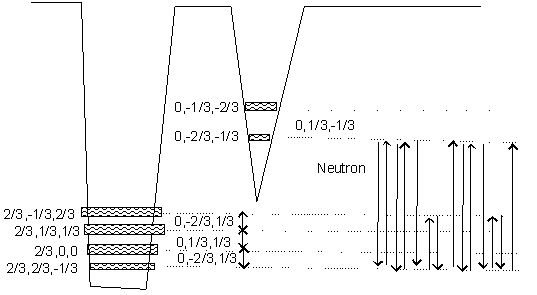Hyperons requires the knowledge of another dephasing parameter from the pions composition shown below: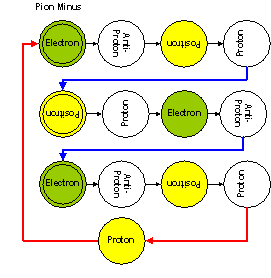Pion Minus diagram.
Here the red lines correspond to the already know half-electron-neutrino. The blue lines correspond to a new dephasing process.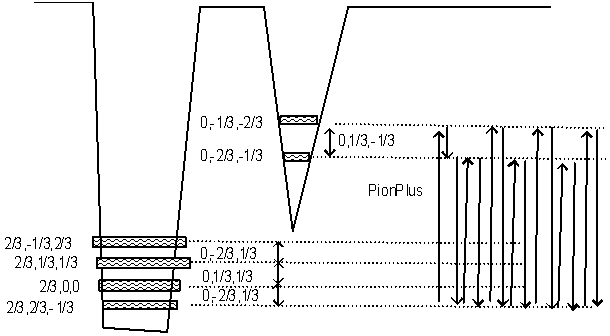This dephasing process happens by rotations on the upper states and corresponds to rotations of the electron state within the 3D Universe. With this dephasing parameter all other particles in the Univers can have their masses calculated. If you know how to split a neutrino, you can make antimatter..:)

Now let's talk about constants. The theory derives formulas for Cosmological Constants like Newton's Gravitational constant G given by: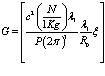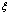= space elasticityP=3.5
K=elasticity anisotropy

Derivation is simple and it is on the pdfs.

The natural laws for Gravitation and Electromagnetism are derived from first principles as: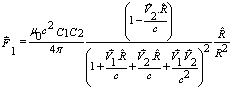orWhere C1 and C2 are the charges traveling at V1 and V2 and c is the speed of light.

For Gravitational one has exactly the same equation (Gyrogravity). This equation is consistent with Pioneer Anomaly , Gravitational Lensing , the Precession of Mercury Perihelion and explains the Galactic Puzzle (the constant angular speed of mass distributions within spiral galaxies). All these demonstrations are posted within this blog.This means that one equation describes Electrostatics, Magnetism and Gravitation and this equation was derived from first principles using the fundamental hypotheses presented above.

The Hypergeometrical Universe theory explains the particle physics aspects of the Universe including the puzzling aspects of Cosmology. Currently there are two inconsistent observations:
1. Hubble measurements using short distance calibration indicates that the Universe is around 14 billion years old.
2. Standard Stellar Candles (Supernovae type 1A) which are supposed to have constant luminosity through all ages of the Universe life, indicates that the furthest observed Stellar Candle to be around 50-60 Billion light years away. This conclusion is arrived by observing exceedingly weak supernovae type 1A explosion. The weaker the supernovae luminosity the larger the distance inferred (apparent luminosity decays with the inverse of the distance squared).

 ActualRadius= ActualAgeOfTheUniverse 21.72 AgeOfTheUniverse as inferred from Hubble Constant=15.36 Pi/180 RadiusOfTheUniverse Lambda RedShit Candle Inferred Distance (BillionLightYears) alpha alpha 45-alpha 1A StellarCandleSize 21.72 1.00 - - 45 0.79 - 1.00 21.34 1.02 (0.02) (0.39) 44 0.77 0.02 0.98 20.95 1.04 (0.04) (0.79) 43 0.75 0.03 0.96 20.56 1.06 (0.05) (1.21) 42 0.73 0.05 0.95 20.15 1.08 (0.07) (1.64) 41 0.72 0.07 0.93 19.75 1.10 (0.09) (2.09) 40 0.70 0.09 0.91 19.33 1.12 (0.11) (2.56) 39 0.68 0.10 0.89 18.91 1.15 (0.13) (3.05) 38 0.66 0.12 0.87 18.49 1.17 (0.15) (3.55) 37 0.65 0.14 0.85 18.06 1.20 (0.17) (4.07) 36 0.63 0.16 0.83 17.62 1.23 (0.19) (4.61) 35 0.61 0.17 0.81 17.18 1.26 (0.21) (5.18) 34 0.59 0.19 0.79 16.73 1.30 (0.23) (5.77) 33 0.58 0.21 0.77 16.28 1.33 (0.25) (6.38) 32 0.56 0.23 0.75 15.82 1.37 (0.27) (7.02) 31 0.54 0.24 0.73 15.36 1.41 (0.29) (7.68) 30 0.52 0.26 0.71 14.89 1.46 (0.31) (8.38) 29 0.51 0.28 0.69 14.42 1.51 (0.34) (9.11) 28 0.49 0.30 0.66 13.95 1.56 (0.36) (9.88) 27 0.47 0.31 0.64 13.47 1.61 (0.38) (10.68) 26 0.45 0.33 0.62 12.98 1.67 (0.40) (11.52) 25 0.44 0.35 0.60 12.49 1.74 (0.42) (12.41) 24 0.42 0.37 0.58 12.00 1.81 (0.45) (13.35) 23 0.40 0.38 0.55 11.51 1.89 (0.47) (14.35) 22 0.38 0.40 0.53 11.01 1.97 (0.49) (15.40) 21 0.37 0.42 0.51 10.51 2.07 (0.52) (16.53) 20 0.35 0.44 0.48 10.00 2.17 (0.54) (17.73) 19 0.33 0.45 0.46 9.49 2.29 (0.56) (19.02) 18 0.31 0.47 0.44 8.98 2.42 (0.59) (20.41) 17 0.30 0.49 0.41 8.47 2.57 (0.61) (21.91) 16 0.28 0.51 0.39 7.95 2.73 (0.63) (23.54) 15 0.26 0.52 0.37 7.43 2.92 (0.66) (25.34) 14 0.24 0.54 0.34 6.91 3.14 (0.68) (27.32) 13 0.23 0.56 0.32 6.39 3.40 (0.71) (29.52) 12 0.21 0.58 0.29 5.86 3.71 (0.73) (32.01) 11 0.19 0.59 0.27 5.33 4.07 (0.75) (34.84) 10 0.17 0.61 0.25 4.81 4.52 (0.78) (38.13) 9 0.16 0.63 0.22 4.28 5.08 (0.80) (42.03) 8 0.14 0.65 0.20 3.74 5.80 (0.83) (46.76) 7 0.12 0.66 0.17 3.21 6.76 (0.85) (52.73) 6 0.10 0.68 0.15 2.68 8.11 (0.88) (60.64) 5 0.09 0.70 0.12 2.14 10.14 (0.90) (71.97) 4 0.07 0.72 0.10 1.61 13.51 (0.93) (90.54) 3 0.05 0.73 0.07 1.07 20.26 (0.95) (131.44) 2 0.03 0.75 0.05 0.54 40.52 (0.98) 1 0.02 0.77 0.02

This inconsistency is easily explained by the Hypergeometrical Universe theory. Just look at the dependence of the Gravitational "constant" G. It decays with the inverse of the Radius of the Universe. This means that at early epochs, when the radius of the Universe was half (quarter) of today's radius (21.72 billion light years as derived from the Pioneer Anomaly results), G would be twice (four) times larger. A larger value of G means that the supernovae 1A will contain a smaller mass in the early Universe. This correction indicates that the perception of 60 Billion Years away supernova, just means that that supernova is at 2.68 billion years old Universe, no paradox there… NO NEED FOR INFLATION….:) whatever price you paid for your condo at the beginning of times, it is the same you would pay now..:)

To understand the reason why there is an angle alpha, one has to remember that one always see the past at 45 degrees angle with respect the the prior hypersphere (prior epoch).  Simple geometry is used to derive the redshift and distance from where we are right now for a given alpha.  The 21.72 billion light years radius of the Universe was derived in the Pioneer Anomaly .  It seems that I called alpha beta in my prior derivation...:) what can I say, this is not my daytime job...:) Look at this plot below and see alpha where it is written beta...:)

This is a live derivation of the hypergeometrical universe theory and I ended up having to tackle the Pioneer anomaly three times before getting everything right.  It doesn't help that the New York subway is so hot and crowded these days...:)

To prove that my theory provides the reason for Quantum Mechanics is trivial and will be presented later in this blog. Only only needs to eliminate the large dilator number approximation (as mentioned many times here and in the pdf containing the theory). This approximation expresses the concept that when one interact large number of dilators in a body, the observed wavelength decreases with the mass (number of dilators). This is the result of the interference pattern along any direction in the neighborhood of a macroscopic body.  This approximation was used to derive the equations for the "Natural Laws", which are macroscopic laws. If you try to use these laws directly in a microscopic problem one wouldn't obtain a quantum mechanical behavior, unless you come up with a contrived theory like QM and an ad-hoc equation like Schroedinger's equation.

When dealing with a single dilator or a small number of dilators as in a molecule, one cannot use this approximation. Not using the approximation means that particles will dance in a four-dimensional lightspeed traveling Lissajous interferometric pattern.  This pattern is imprinted on space itself as dilaton waves interference.

If you want to understand the Cosmogenesis, please let me know..:) The explanation is simple and based upon these two plots..:)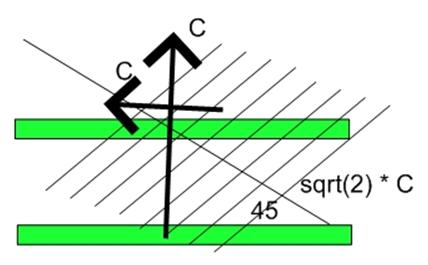Before I forget:
SPACE

What is space. Space and Absolute time are quasi-constants, that is, they exist always as they are now, but they exist after the dimensional transition that created the extra four spatial dimensions..:)

I am agnostic with respect to the proposition that space is being created ahead of the hypergeometrical universe expansion or if there is already space there...:)  That is irrelevant because it cannot be tested.

Space itself, as far as I am concerned, is just a Cartesian medium which presents no friction, no dephasing events and has a restoring force (elasticity). I am agnostic with respect to any sub-structure since I don't believe any experiment could probe any substructure. The reason is that colliding particles also follow the Quantum Lagrangian Principle, that is, they are always in quantized positions determined by the interference pattern of the dilaton field during their interactions.

A cartesian space would work for all two cross-sections if I didn't have to reproduce current relativistic views.  Current views based upon the choice of dynamic laws requires TauXYZ (spacetime) to be hyperbolic, thus the hyperbolic tangent in the Hypergeometrical Universe Newton's Second Law.

Huygens Principle

Huygens and Newton debated how to describe the dynamics of interacting bodies. Newton won.

Huygens was biased by his optical interference experiments and wanted to explain all motions (interaction) as being the sole product of interacting waves, a simplistic reasoning. If you think that the Quantum Lagrangian Principle is equivalent to the Huygens Principle you are wrong. The QLP describes what is happening on the RXYZ cross-section shown in the first figure in this posting. We don't live there…:) at least, we can only see that cross-section with the eye of the mind..:)

We live in the TauXYZ cross-section, where Tau is the proper time. I modified Newton's Second Law to present it as a Spatial Stress-Strain relationship between these two cross-sections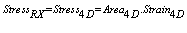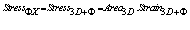Let's make Area 4D =1 then Area3D is just the overlapping of the unitary dilator 4D volume with the Fabric of Space.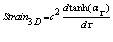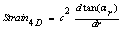Notice the hyperbolic tangent on the 3D Strain. This is a requirement for relativity.

Even though the dilator moves in the RXYZ following an interference pattern, the dynamics has to be described in the TauXYZ. In that cross-section, wavelengths vary with velocity and one cannot simply imagine motion through an interference pattern. Here one has to take into consideration Doppler shifts of the dilator wavelength while creating the 3D Lissajous figures where dilators will pop-up at each de Broglie step of the Universe expansion. Of course, these Doppler shifts are measured in the Schroedinger equations as changes in momentum or forces.

Had Huygens followed this simple reasoning, he would have discovered Quantum Mechanics.

Cheers,

MP
Currently unrated

### New Comment

required

required (not published)

optional

required